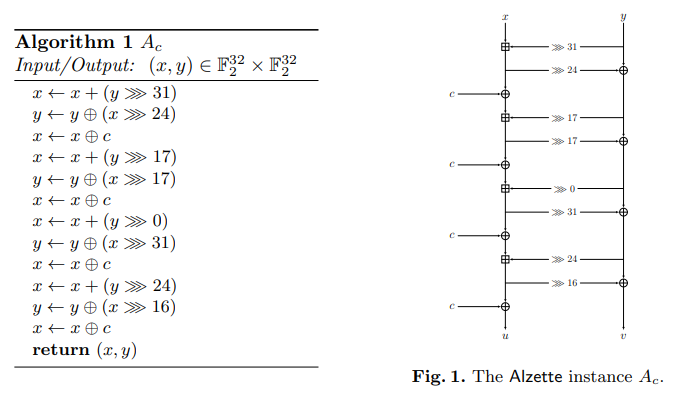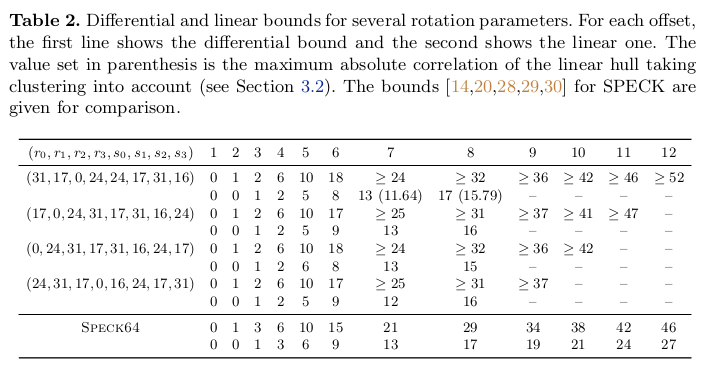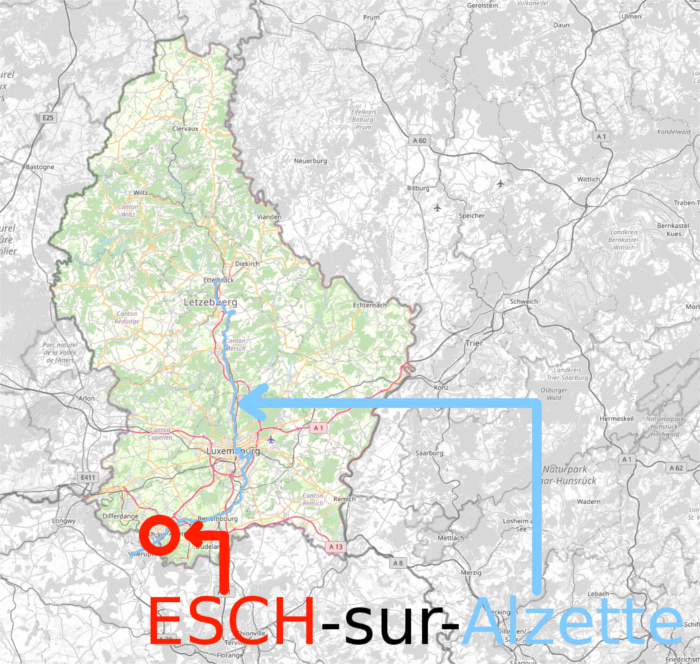# Alzette, an ARX-box

Alzette is a 64-bit ARX-based S-box, which can be evaluated in constant time using only 12 instructions on modern CPUs. Its parallel application can also leverage vector (SIMD) instructions. One iteration of Alzette has differential and linear properties comparable to those of the AES S-box, and two are at least as secure as the AES super S-box. As the state size is much larger than the typical 4 or 8 bits, the study of the relevant cryptographic properties of Alzette was not trivial and required extensive computer experiments.

Alzette is parametrized by a 32-bit constant $$c$$, used 4 times in the structure. The figures below represent the Alzette structure. We remind that the addition is done modulo $$2^{32}$$.For example, the following constants for Alzette are used in the Sparkle permutation:

$c_0 = \mathtt{b7e15162}, c_1 = \mathtt{bf715880}, c_2 = \mathtt{38b4da56}, c_3 = \mathtt{324e7738},$ $c_4 = \mathtt{bb1185eb}, c_5 = \mathtt{4f7c7b57}, c_6 = \mathtt{cfbfa1c8}, c_7 = \mathtt{c2b3293d}.$

Alzette has the following advantages:

• it relies on 32-bit rather than 16-bit operations, meaning that (according to [Triathlon, Sect. 5]) it is suitable for a larger number of architectures;
• it makes better use of barrel shift registers (when available) and has more efficient rotation constants (for platforms on which they have different costs);
• its differential and linear properties are superior to those of a scaled-up Sparx ARX-box;
• our analysis takes more attacks into account, and is confirmed experimentally whenever possible.

## Primitives using Alzette

Alzette is one of the main building blocks of the Sparkle-suite: it is the main source of nonlinearity and cryptographic strength in the Sparkle permutation. Furthermore, it was naturally used to construct a classic block cipher CRAX and a tweakable block cipher TRAX.

## Implementation Properties

Alzette can be executed in only 12 cycles on a 32-bit ARM Cortex-M3 and 122 cycles on an 8-bit AVR ATmega128 processor. Besides, the code size is low: respectively 24 and 176 bytes on those platforms.

## Security Properties

The rotation constants were chosen carefully in a way that maximizes security and efficiency. While each rotation has the same cost in 32-bit ARM processors (i.e., 0 because rotation is for free on top of XOR, resp., AND), we further aimed for minimizing the cost with regard to 8-bit and 16-bit architectures. For security properites, we aimed to minimize the provable upper bound on the expected differential trail probability (resp. expected absolute linear trail cor- relation) of a differential (resp. linear) trail. The final rotation constants together with the respective bounds (for different offsets, i.e., starting rounds) are summarized in the following table.Besides having strong resistance against linear and differential attacks, Alzette was checked for invariant subspaces, nonlinear invariants, bit-based division property propagation, and linearization attacks. The analysis showed that these attacks methods have little strength against Alzette. For more details, we refer to the documentation (extended Alzette paper, Sparkle-suite specification).

## On the Names

Esch is the name of a city in Luxembourg that is very close to the campus of the University of Luxembourg, and lies on the Alzette river.